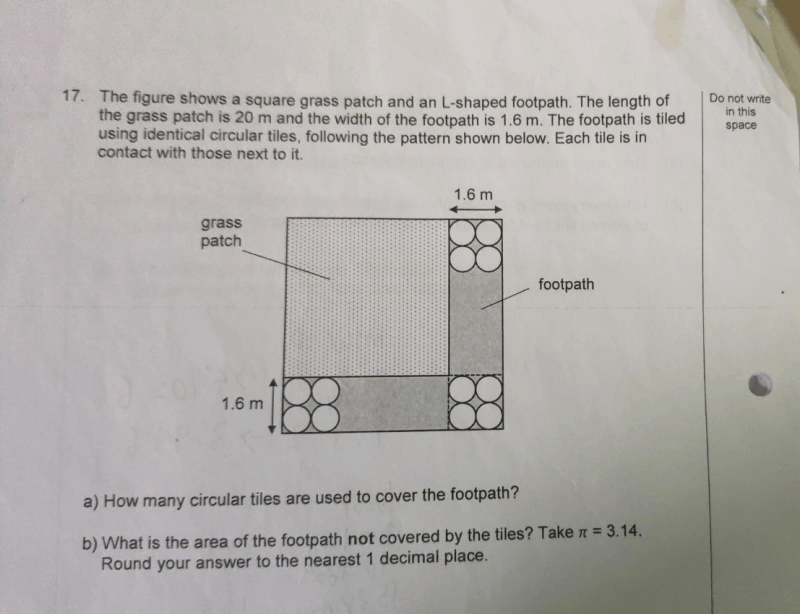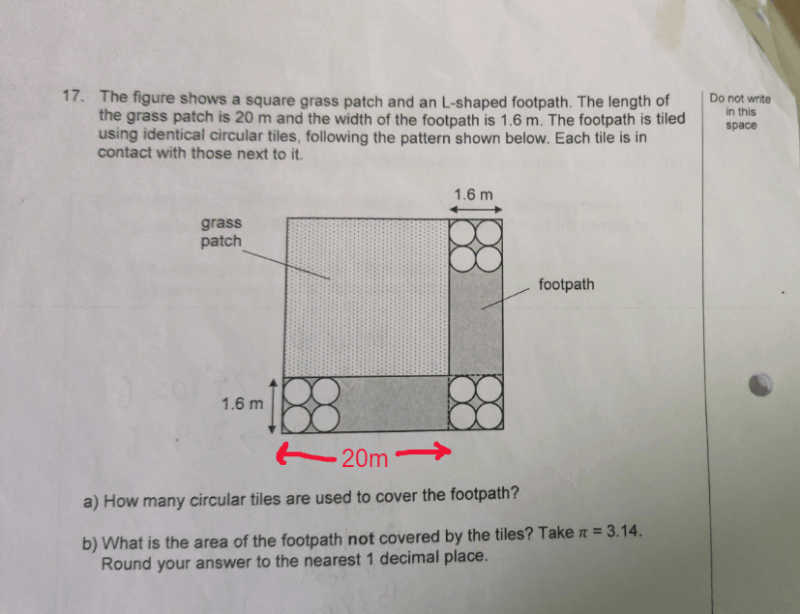# QuestionDiameter of each circular tile is (1.6 ÷ 2) = 0.8m

Number of tiles to cover 20m -> 20 ÷ 0.8 = 25

Since the path is 1.6m wide, (25 × 2) = 50 tiles needed to cover the 20m × 1.6m path.

Since there are 2× of such path, (50 × 2) = 100 tiles

Finally, there are 4 tiles at the corner.

(a) Number of tiles used -> 104

Area of footpath -> 2 × (20 × 1.6) + (1.6 × 1.6) = 66.56 m²

Area of 104 tiles -> 104 × (3.14 × 0.4 × 0.4) = 52.2496 m²

(b) Area not covered -> 66.56 – 52.2496 = 14.3 m² (to nearesr 1 decimal place)

0 Replies 0 Likes